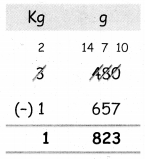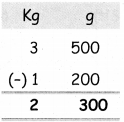Tamilnadu State Board New Syllabus Samacheer Kalvi 4th Maths Guide Pdf Term 2 Chapter 4 Measurements Ex 4.1 Textbook Questions and Answers, Notes.

## Tamilnadu Samacheer Kalvi 4th Maths Solutions Term 2 Chapter 4 Measurements Ex 4.1

Question 1.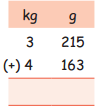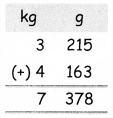Question 2.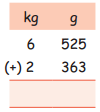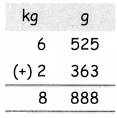Question 3.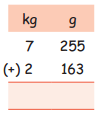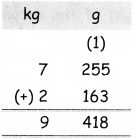2. Subtract

Question 1.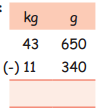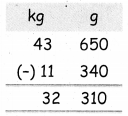Question 2.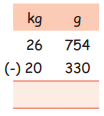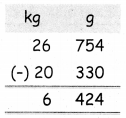Question 3.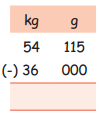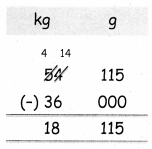Question 3.
Raman bought 3 kg 250 g of tomatoes, 5 kg 110 g of potatoes and 3 kg 750 g of onions. What is the total weight of the vegetables ?
Weight of Tomatoes = 3 kg 250 g
Weight of Potatoes = 5 kg 110 g
Weight of Onions = 3 kg 750 g
∴ Total weight of vegetables = 3 kg 250 g + 5 kg 110 g + 3 kg 750 g
= 12 kg 110 g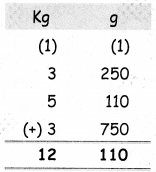Ramana bought 12 kg 110 g of vegetablesQuestion 4.
Kannan bought some fruits and vegetables whose total weight is 3 kg 480 g. If the weight of fruits is 1 kg 657 g, find weight of the vegetables.